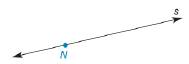Chapter 1.6, Problem 5E### Elementary Geometry for College St...

6th Edition
Daniel C. Alexander + 1 other
ISBN: 9781285195698

#### Solutions

Chapter
Section### Elementary Geometry for College St...

6th Edition
Daniel C. Alexander + 1 other
ISBN: 9781285195698
Textbook Problem
1 views

# In Exercise 5 to 9, use a compass and a straightedge to complete the constructions. Given: Point N on line s Construct: Line m through N so that m ⊥ sTo determine

To find:

The complete construction of Line m through N so that ms.

Explanation

Given:

A point N is marked on the line s.

Construction:

A point N is marked on the line s.

The steps are given below for the complete construction.

a) Use the point N as center and draw the arcs of any equal radius on the given line s.

b) Mark the intersection points as A and B.

c) Use A and B as the centers for the compass and take the radius greater than the length of AN.

d) Draw new arcs above and below the lines from A and B

### Still sussing out bartleby?

Check out a sample textbook solution.

See a sample solution

#### The Solution to Your Study Problems

Bartleby provides explanations to thousands of textbook problems written by our experts, many with advanced degrees!

Get Started

#### In Exercises 2340, find the indicated limit. 24. limx23

Applied Calculus for the Managerial, Life, and Social Sciences: A Brief Approach

#### Convert the expressions in Exercises 6584 to power form. 8

Finite Mathematics and Applied Calculus (MindTap Course List)

#### Find for y defined implicity by .

Study Guide for Stewart's Multivariable Calculus, 8th